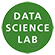Section for Statistics and Probability Theory

Research

The research in the section covers topics ranging from probability theory and mathematical statistics to applied statistics. Main themes include Markov processes, biostatistics, biomathematics, inference for statistical models based on stochastic processes, general principles for statistical inference, graphical models and their applications (see homepage), asymptotic statistical theory, functional data analysis, and applications of statistical methodology and stochastic processes in bioinformatics, neuroscience, systems biology, reaction networks (see homepage), physiology, and earth science.

In particular, the section works with statistical inference from discrete time sampling of continuous time processes, such as models given by stochastic differential equations and jump processes, or from first-passage-time data from these models. Also, statistical learning and inference for point processes are studied, as well as Monte Carlo simulation, hidden Markov models. Especially gene expression data, DNA data and stochastic neuronal models are studied.

Visit the research groups Mathematics of reaction networks (MBIO) and Copenhagen Causality Lab (CoCaLa).

The section is part of Copenhagen Statistics Network.The section hosts Data Science Laboratory together with the Department for Computer Science. DataScienceLab develops and applies statistical methods in close collaboration with researchers from other departments at the faculty.

Study programme

Prospective students are encouraged to visit the study websites in Danish or English.

Current students can visit the webpage MCs in Statistics on the student intranet.